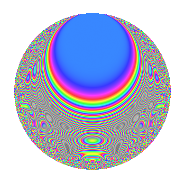# Properties

 Label 403.2.rLevel 403 Weight 2 Character orbit r Rep. character $$\chi_{403}(218,\cdot)$$ Character field $$\Q(\zeta_{6})$$ Dimension 68 Newforms 1 Sturm bound 74 Trace bound 0

# Related objects

## Defining parameters

 Level: $$N$$ = $$403 = 13 \cdot 31$$ Weight: $$k$$ = $$2$$ Character orbit: $$[\chi]$$ = 403.r (of order $$6$$ and degree $$2$$) Character conductor: $$\operatorname{cond}(\chi)$$ = $$13$$ Character field: $$\Q(\zeta_{6})$$ Newforms: $$1$$ Sturm bound: $$74$$ Trace bound: $$0$$

## Dimensions

The following table gives the dimensions of various subspaces of $$M_{2}(403, [\chi])$$.

Total New Old
Modular forms 80 68 12
Cusp forms 72 68 4
Eisenstein series 8 0 8

## Trace form

 $$68q + 32q^{4} - 34q^{9} + O(q^{10})$$ $$68q + 32q^{4} - 34q^{9} + 8q^{10} - 12q^{11} - 16q^{12} + 6q^{13} - 8q^{14} - 36q^{16} - 6q^{17} + 12q^{19} - 12q^{20} - 20q^{22} - 8q^{23} + 48q^{24} - 72q^{25} - 12q^{27} - 6q^{28} + 32q^{30} + 6q^{33} + 30q^{35} + 40q^{36} - 42q^{37} - 36q^{38} - 14q^{39} + 8q^{40} + 18q^{41} - 16q^{42} + 12q^{43} + 60q^{45} + 30q^{46} - 46q^{48} + 22q^{49} + 56q^{51} + 20q^{53} - 114q^{54} - 6q^{55} - 2q^{56} - 12q^{58} + 6q^{59} + 6q^{61} - 8q^{62} - 30q^{63} + 24q^{64} + 24q^{65} + 8q^{66} - 48q^{67} + 58q^{68} - 28q^{69} - 30q^{71} + 72q^{72} + 8q^{74} - 4q^{75} - 12q^{76} - 20q^{77} + 26q^{78} + 16q^{79} + 42q^{80} - 58q^{81} - 42q^{82} - 72q^{84} + 30q^{85} - 20q^{87} + 64q^{88} + 18q^{89} + 52q^{90} - 22q^{91} + 48q^{92} + 8q^{94} - 32q^{95} - 168q^{98} + O(q^{100})$$

## Decomposition of $$S_{2}^{\mathrm{new}}(403, [\chi])$$ into irreducible Hecke orbits

Label Dim. $$A$$ Field CM Traces $q$-expansion
$$a_2$$ $$a_3$$ $$a_5$$ $$a_7$$
403.2.r.a $$68$$ $$3.218$$ None $$0$$ $$0$$ $$0$$ $$0$$

## Decomposition of $$S_{2}^{\mathrm{old}}(403, [\chi])$$ into lower level spaces

$$S_{2}^{\mathrm{old}}(403, [\chi]) \cong$$ $$S_{2}^{\mathrm{new}}(13, [\chi])$$$$^{\oplus 2}$$